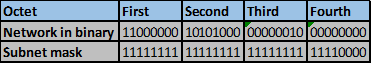# Addressing in ipv4 – part 1

## Overview

In the previous chapter, we looked at the network layer and its involvement in communication. in this chapter, we will look at ipv4 addressing. This is one of the most important concepts in networking and will be critical in your overall success in networking. Understanding this chapter is critical to the rest of your studies. This chapter is divided into two parts so that it can better understood. In the first part, we will look at binary vs decimal, then we will be introduced to subnetting. Then we will finish up by subnetting a few addresses.

## Decimal vs binary

We are used to decimals. The number systems used everywhere today is mostly binary. Base of 10.

10^0=1

10^1=10

10^2=100

This is what we use in day to day activities. However, computers are limited to only 2 digits. “1” and “0”, which represent “ON” and “OFF”. The ip addressing system we use is based on this concept. All ipv4 addresses that we use are a decimal representation of the binary form that the computer knows about.

The table below shows the difference between decimal and binary connotations.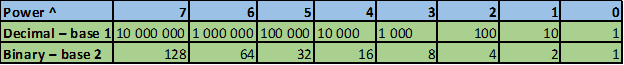You will be seeing the binary table more frequently as we continue in this chapter and others.

I believe the most of you have seen an ip address. The ip addressing scheme used contains two parts.

• The network part – from the left hand side
• The host part – from the right hand side

In IPv4 addresses, the high-order bits (bits starting from the left side) represent the network address, the low-order bits (bits on the right hand side) represent the host portion. In IPv4 addressing, hosts who have matching high-order bits are said to be in the same network.

The number of bits in each side either the network portion or the hosts portion determine the number of networks and hosts respectively.

To understand how networks work, we need to understand binary to decimal and decimal to binary conversion.

Take 192.168.1.0 as the ip address example. We need to convert this to binary. The figure shown below shows how this is done.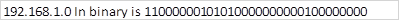The figure below shows how this can be done.

Keep these steps in mind:

1. Divide the 32 bits into 4 octets.
2. Convert each octet to decimal.
3. Add a “dot” between each decimal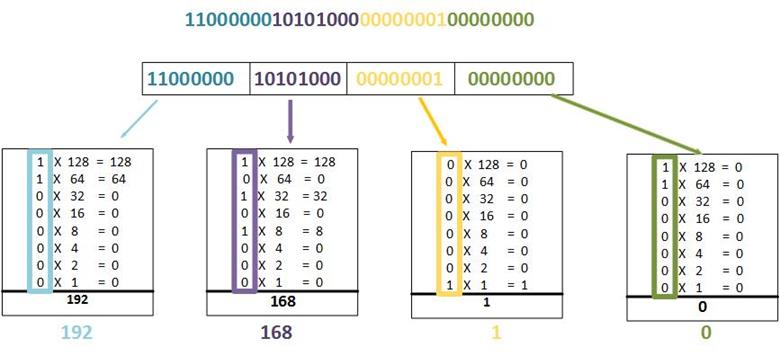NOTE: you need to practice converting decimal form addresses to binary form for you to understand IPv4 addressing, therefore, practice is vital.

Now that we have learnt this concept, the table below shows a way you can convert each number into binary easily.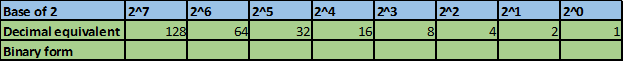Based on this table, you can convert any number to binary or decimal easily.

For example, if we want to convert 255 to binary, we would take the following steps.

Take 255, compare it to 128, if it is larger, subtract 128 to give 127

Is 127 larger than 64 if yes then subtract 64 to give 63

is 63 larger than 32, if yes subtract 32 to give 31

is 31 larger than 16, if yes subtract 16 to give 15

is 15 larger than 8, if yes subtract 8 to give 7,

is 7 larger than 4, if yes subtract 4 to give 3

is 3 larger than 2, if yes then subtract 2 to give 1

is 1 larger or equal to 1, yes. This is the last step of the process.

This can be summarized in the flowchart shown below.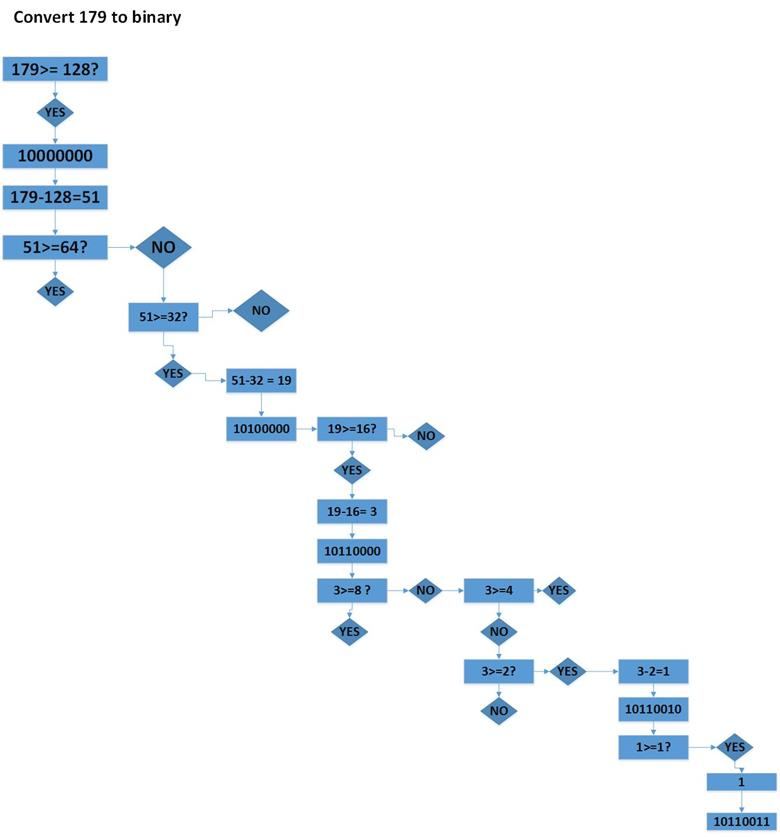Now if a number from the example above is larger or equal to the binary equivalent, the binary number will be 1, if it is less than that then the binary will be 0.

Therefore, the binary conversion of 255 will be, 11111111.

Now based on these two forms, can you convert the following to binary?

178, 168, 150, 10, 4.

These sequential steps will be useful in knowing how to subnet.

Because our representation of addresses is limited to decimal values for a single octet, we will only examine the process of converting 8-bit binary to the decimal values of 0 to 255.

In any IPv4 network address range, we have 3 main types of addresses:

• Network address – this is the address we use to name the network.
• Broadcast address – this is a special type of IPv4 address that is used to send packets to all hosts in a particular network
• Host addresses – these addresses can be assigned to hosts or devices in our networks such as PCs, laptops, IP phones among others.

The network address is the name we give to a network, for example a network with the address shown below is known as “the 10.0.0.0” network. All hosts in this network belong to the 10.0.0.0 network. The network portion for these hosts would all be the same.

In networking the first IPv4 address in a network is usually the network address.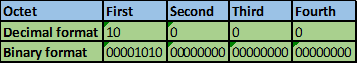The broadcast address in IPv4 is a special address that is used to send information to all the hosts in a network. The broadcast address is always the highest or last address in a network. In the example below, the broadcast address is the address with all 1’s in the host region.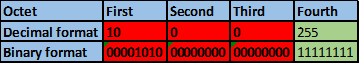The first three octets represent the network portion, the third represents the broadcast address.

As we discussed earlier, the hosts in our networks need to be assigned an IPv4 address. The address that can be assigned to these hosts is any IP address that falls in between the network IP address and the broadcast IP address.

NOTE: you cannot assign the network and broadcast address to a host and these are reserved.

From the above, we can ask ourselves, how can we tell how many bits represent the network and host portion? Well, for this, we use a prefix length. This is done by counting the number of bits in the network portion. When we represent the address in decimal form, we use a back slash “/” followed by the number of high-order bits as shown in the table below.

In this case the network 172.16.1.0 has 24 high-order bits and therefore is represented as 172.16.1.0/24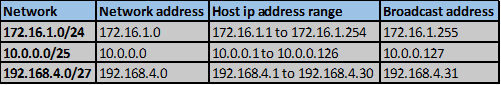The number of hosts on the network or the number of network bits determine the prefix number to be used.

NOTE: when the number of high-order bits increases, the number of low-order bits decreases, and therefore less addresses for hsots.

The formula to get the number of hosts per prefix is

2^n-2= host addresses, where n is the number of host bits.

So for 192.168.3.0/28 the number of network bits are 28, since ipv4 addresses have 32 bit, 4 bits will make up the host portion.

From the above formula, the hosts in this network will be:

2^n-2= hosts in the network

2^4-2= 14

Therefore there will be 14 host IP addresses.

As we saw earlier, the IPv4 address is divided into the network and host portions. We have also said that the prefix length is the number of bits in the network portion or high order bits. And it tells us the number of host IP addresses and networks.
The routers and other devices in the network also need to know the number of high-order bits and low-order bits, for this they use the subnet mask which is a representation of the prefix length in decimal. As we mentioned earlier, the prefix length is the number of 1’s from the left hand side and it denotes the network and the host portion.

To represent the prefix length in network devices use a special type of IPv4 address known as the subnet mask. It is made up of 32 bits just like in the regular IPv4 address. The subnet mask is shown by placing a 1 in the network portion and a 0 in the host portion. For example, a /24 prefix is expressed as a subnet mask as 255.255.255.0 (11111111.11111111.11111111.00000000). The remaining bits (low order) of the subnet mask are zeroes, indicating the host address within the network.

When we configure the IP address on the network devices, it must be accompanied by the subnet mask.

## Binary ANDing

So the question is, how does the router know to which network an ip address from a packet it just received belongs to, when making it’s forwarding decision?

The IPv4 host address is logically ANDed with its subnet mask to determine the network address to which the host is associated. When this ANDing between the address and the subnet mask is performed, the result yields the network address.

### The AND Operation

ANDing is a logic operation, the ANDing process is used to compute the network address, given the subnet mask and an IP address. The Logical ANDing operation gives the following results when the comparison is made on an IP address and subnet mask bits.

1. 1 AND 1 = 1
2. 1 AND 0 = 0
3. 0 AND 1 = 0
4. 0 AND 0 = 0

The reasons for binary ANDing are:

• Network devices in one network can decide whether the packet belongs to the local network or it should be forwarded to a remote network
• The router bases its forwarding decisions on the results of the binary ANDing process.

The table below shows the ANDing process for the network 192.168.2.45/24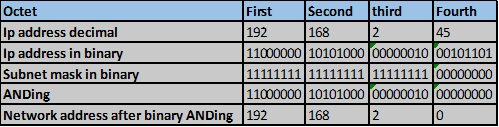Therefore, the network address for the Ip address 192.168.2.45/24 would be 192.168.2.0

You should learn binary ANDing by practicing. The ip addresses shown below would be a good place to start.

Do you think you can determine the network addresses of the following ip addresses?

• 192.168.1.35/27
• 10.10.10.23/14
• 172.16.3.134/19

So now that we understand how to convert ip addresses from decimal to binary, it is time to introduce subnetting.

Subnetting allows us to create more networks from a single ip address block. These networks are interconnected using routers and therefore each interface on a router has to be on a different network.

NOTE: the networks we will create through subnetting will be referred to as subnets. Therefore you should not get confused when you see the word subnet instead of network.

Subnetting is a simple process where we borrow bits from the host portion in order to create new networks. Take the example shown in the figure below. The address 192.168.1.0/24 is the major class, if we were to borrow 2 bits from the hosts portion, the new prefix length would be 26. Therefore, the 2 bits would be assigned to the network portion.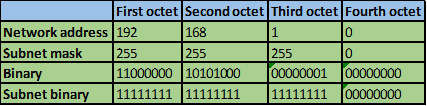When we borrow the host bits, the new binary address would be the same, however, the subnet mask which shows the network portion will be different as shown below: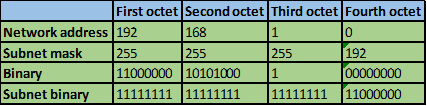When we extend the subnet mask by borrowing the bits in the host portion, we essentially double the number of networks. However, the more bits we borrow from the host portion, the less the number of host IP addresses available per subnet that is created.

The formula for calculating how many subnets are created whenever we borrow host bits is:

2^n= number of new networks.

To calculate the number of hosts per network, we use the formula of

2^n – 2 where n = the number of bits left for hosts.

Take the example shown below of network 172.16.1.0/24, if we want to subnet this network to give 2 networks we would need to borrow 1 host bits from the host side and add them to the network portion.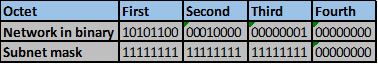After borrowing the two host bits from the fourth octet, the new network will be as shown below.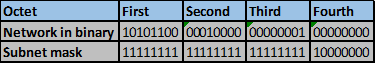The subnet mask would have 1 more 1 bits.

Therefore after borrowing 1 host bits we will have created 2 more networks but we will have decreased the number of hosts.

Now the new subnets would be as shown below

172.16.1.0/25172.16.1.128/25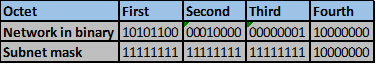The subnet mask would be 255.255.255.128

NOTE: whenever we subnet, the last subnet will always have the same bits as the last octet of the new subnet mask.

Take the example shown below.

If we subnet 172.16.1.0/24 to give 8 new networks.

Step 1. How many bits do we need to borrow from the hosts segment?

2^n=8

2^3=8

Therefore we need to borrow 3 host bits.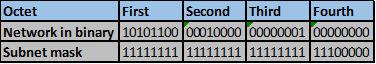The first 2 and the last subnets will be:

172.16.1.0/27172.16.1.32/27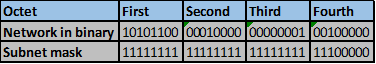Last subnet – 172.16.1.224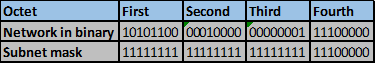The increment number is usually the last borrowed host bit. In the above scenario, this is 32.

The subnets for 172.16.1.0/24 after subnetting to /27 will be: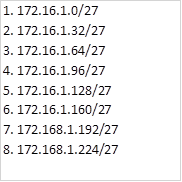## Why subnet

In our networks, the IP address requirements may vary. We may need few ip addresses for connections such as point-to-point, we may have a department that has 200 users while another only has 20.

Subnetting allows us to divide the networks so as to optimally use the ip addresses. If we assigned 200 ip addresses to a network that only needs 20, we would be wasting very many addresses.

If we use an analogy of a telephone service provider, not subnetting would be like giving a customer 100 telephone numbers whilst they only require 1.

When subnetting, we should carefully consider expansion plans since our networks may grow due to demand.

In the scenario shown below, we will subnet based on the number of HOSTS. This is a crucial element in understanding subnetting.

## The scenario

In the scenario below, we are expected to subnet three network addresses:

172.16.1.0/24 for LAN A

172.16.2.0/24 for LAN B

192.168.2.0/24 for LAN C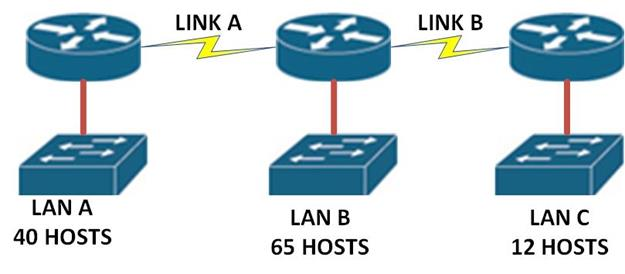So we will begin with the WAN links.

We need to calculate how many right hand side bits we need, then the rest will be allocated to the network portion.

2^n=2 – 2

2^2=4

4-2 = 2

Therefore, we only need 2 host bits. 6 bits will be in the network portion.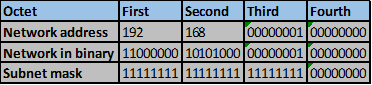The new subnet mask after we add 6 bits to the network portion will be: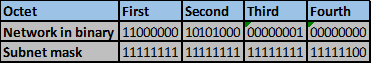192.168.1.0/30

Therefore, the increment will be 4. In this case the first network will be 192.168.1.0/30The second network will be 192.168.1.4/30 as shown below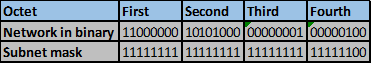### LAN A

For part 2 we need to provide enough host ip addresses for LAN A.

LAN A requires 40 ip addresses.

Therefore, we need to calculate how many host bits we need to accommodate this.

2^n-2= 40

2^5- 2=30, this is less than 40 therefore it will not be adequate.

2^6-2 = 62. This therefore will be enough for the hosts in LAN A.

The host bits will be 6 and we will borrow 2 host bits to get these new networks.

The new subnet mask for the 172.16.1.0/24 network after subnetting will be: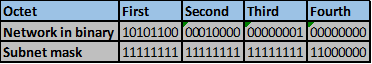255.255.255.192

The increment number will be 64, therefore the four networks will be: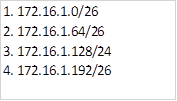### LAN B

We need 65 host ip addresses, given the network 172.16.2.0/24

First, we need to determine how many host bits will be needed in the hosts portion.

2^n-2= 65

2^6-2=62, which will not be enough.

2^7-2= 126, which will be enough and will allow for expansion.

Therefore, the hosts portion will have 7 bits and the network portion will borrow 1 bit from the fourth octet.

The new subnet mask for the network 172.16.2.0 will be as shown below.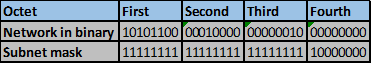This is 255.255.255.128

Therefore, the increment will be 128.

The first network will be as shown below. 172.16.2.0/25The second and last network will be 172.16.2.128/25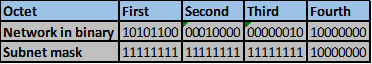### LAN C

In LAN C, we need to have subnets that will accommodate 12 hosts per subnet using network 192.168.2.0/24.

First we determine how many host ip address bits will be needed.

2^n-2=12

2^4-2=14 will be enough.

Therefore, the host portion will have 4 bits, while the network segment will borrow 4 bits.

The new subnet mask for the network 192.168.2.0 will be.